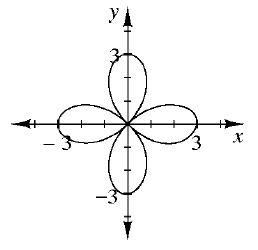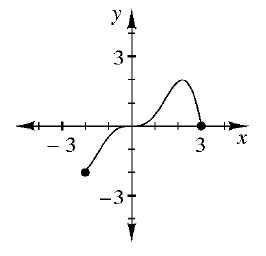### Home > CCA2 > Chapter Ch4 > Lesson 4.1.3 > Problem4-45

4-45.

Examine the graph of each relation below. For each part below, decide if the relation is a function and then state the domain and range.

1.For a relation to be a function, each input can only have one output. That is, each $x$-value can only have one corresponding $y$-value.

1.